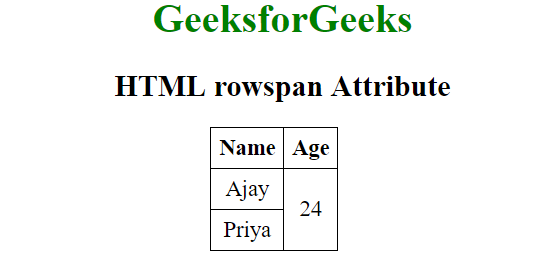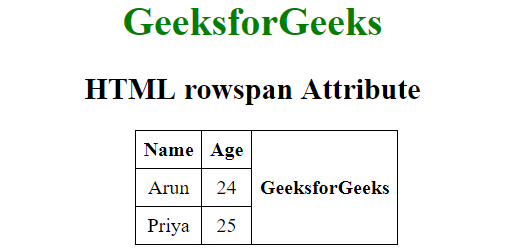# HTML | rowspan Attribute

The rowspan attribute in HTML specifies the number of rows a cell should span. That is if a row spans two rows, it means it will take up the space of two rows in that table. It allows the single table cell to span the height of more than one cell or row. It provides the same functionality as “merge cell” in the spreadsheet program like Excel.
Usage: It can be used with <td> and <th> element in an HTML Table.

Attribute Values: It contains a value i.e number Which specify the number of rows that a table cell should span.

• <td>: The rowspan attribute when used with <td> tag determines the number of standard cells it should span.
Syntax:
`<td rowspan = "value">table content...</td>`

The value specifies the number of rows that the cell fills. The value must be a integer.
Example:

## html

 ` ` `<``html``> ` `    ``<``head``> ` `        ``<``title``>HTML rowspan Attribute ` `        ``<``style``>` `            ``table, th, td {` `                ``border: 1px solid black;` `                ``border-collapse: collapse;` `                ``padding: 6px;` `            ``}` `        ``` `    `` `   `    ``<``body` `style` `= ``"text-align:center"``> `   `        ``<``h1` `style` `= ``"color: green;"``>GeeksforGeeks` `        ``<``h2``>HTML rowspan Attribute`   `        ``<``table``>` `            ``<``tr``>` `                ``<``th``>Name` `                ``<``th``>Age` `            ``` `            ``<``tr``>` `                ``<``td``>Ajay` `                ``` `                ``<``td` `rowspan``=``"2"``>24` `            ``` `            ``<``tr``>` `                ``<``td``>Priya` `            ``` `        ``` `        `  `    `` ` `                    `

Output:• <th>: The rowspan attribute when used with <th> tag determines the number of header cells it should span.
Syntax:
`<th rowspan = "value">table content...</th>`

The value specifies the number of rows that the cell fills. The value must be a integer.
Example:

## html

 ` ` `<``html``> ` `    ``<``head``> ` `        ``<``title``>HTML rowspan Attribute ` `        ``<``style``>` `            ``table, th, td {` `                ``border: 1px solid black;` `                ``border-collapse: collapse;` `                ``padding: 6px;` `            ``}` `        ``` `    `` ` `    `  `    ``<``body` `style` `= ``"text-align:center"``> ` `        ``<``h1` `style` `= ``"color: green;"``>GeeksforGeeks` `        ``<``h2``>HTML rowspan Attribute` `        `  `        ``<``table``>` `            ``<``tr``>` `                ``<``th``>Name` `                ``<``th``>Age` `                ``` `                ``<``th` `rowspan``=``"3"``>GeeksforGeeks` `            ``` `            ``<``tr``>` `                ``<``td``>Arun` `                ``<``td``>24` `            ``` `            ``<``tr``>` `                ``<``td``>Priya` `                ``<``td``>25` `            ``` `        ``` `    `` ` `                    `

Output:Supported Browsers: The browser supported by rowspan attribute are listed below: